Next: Numerical Methods
Up: EXPLANATION AND EXAMPLES
Previous: Radial Velocity and Light-Time Corrections

## Focal-Plane Astrometry

The relationship between the position of a star image in the focal plane of a telescope and the star's celestial coordinates is usually described in terms of the tangent plane or gnomonic projection. This is the projection produced by a pin-hole camera and is a good approximation to the projection geometry of a traditional large f-ratio astrographic refractor. SLALIB includes a group of routines which transform star positions between their observed places on the celestial sphere and their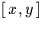coordinates in the tangent plane. The spherical coordinate system does not have to be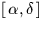but usually is. The so-called standard coordinates of a star are the tangent plane, in radians, with respect to an origin at the tangent point, with the y-axis pointing north and the x-axis pointing east (in the direction of increasing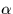). The factor relating the standard coordinates to the actualcoordinates in, say, millimetres is simply the focal length of the telescope.

Given theof the plate centre (the tangent point) and theof a star within the field, the standard coordinates can be determined by calling sla_S2TP (single precision) or sla_DS2TP (double precision). The reverse transformation, where theis known and we wish to find the, is carried out by calling sla_TP2S or sla_DTP2S. Occasionally we know the both theand theof a star and need to deduce theof the tangent point; this can be done by calling sla_TPS2C or sla_DTPS2C. (All of these transformations apply not just tobut to other spherical coordinate systems, of course.) Equivalent (and faster) routines are provided which work directly in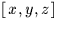instead of spherical coordinates: sla_V2TP and sla_DV2TP, sla_TP2V and sla_DTP2V, sla_TPV2C and sla_DTPV2C.

Even at the best of times, the tangent plane projection is merely an approximation. Some telescopes and cameras exhibit considerable pincushion or barrel distortion and some have a curved focal surface. For example, neither Schmidt cameras nor (especially) large reflecting telescopes with wide-field corrector lenses are adequately modelled by tangent-plane geometry. In such cases, however, it is still possible to do most of the work using the (mathematically convenient) tangent-plane projection by inserting an extra step which applies or removes the distortion peculiar to the system concerned. A simple r1=r0(1+Kr02) law works well in the majority of cases; r0 is the radial distance in the tangent plane, r1 is the radial distance after adding the distortion, and K is a constant which depends on the telescope (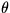is unaffected). The routine sla_PCD applies the distortion to anand sla_UNPCD removes it. Forin radians, K values range from -1/3 for the tiny amount of barrel distortion in Schmidt geometry to several hundred for the serious pincushion distortion produced by wide-field correctors in big reflecting telescopes (the AAT prime focus triplet corrector is about K=+178.6).

SLALIB includes a group of routines which can be put together to build a simple plate-reduction program. The heart of the group is sla_FITXY, which fits a linear model to relate two sets ofcoordinates, in the case of a plate reduction the measured positions of the images of a set of reference stars and the standard coordinates derived from their catalogue positions. The model is of the form:

xp = a + bxm + cym

yp = d + exm + fym

where the p subscript indicates predicted'' coordinates (the model's approximation to the ideal expected'' coordinates) and the m subscript indicates measured coordinates''. The six coefficients a-f can optionally be constrained to represent a solid body rotation'' free of any squash or shear distortions. Without this constraint the model can, to some extent, accommodate effects like refraction, allowing mean places to be used directly and avoiding the extra complications of a full mean-apparent-observed transformation for each star. Having obtained the linear model, sla_PXY can be used to process the set of measured and expected coordinates, giving the predicted coordinates and determining the RMS residuals in x and y. The routine sla_XY2XY transforms oneinto another using the linear model. A model can be inverted by calling sla_INVF, and decomposed into zero points, scales, x/y nonperpendicularity and orientation by calling sla_DCMPF.Next: Numerical Methods
Up: EXPLANATION AND EXAMPLES
Previous: Radial Velocity and Light-Time Corrections

SLALIB --- Positional Astronomy Library